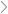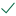Questions & AnswersOperations ManagementTo simulate inventory management for a particular product, assume...

QuestionAnswered step-by-step

# To simulate inventory management for a particular product, assume...

To simulate inventory management for a particular product, assume that the per period demand is an integer value quantity which follows the normal distribution with mean and standard deviation 50 and 15, respectively. If the demand exceeds the starting inventory, then the ending inventory would be zero, that is, the 'extra" demand is lost. Therefore, the ending inventory level is never negative. Ordering will take place at the end of the period, based on the ending inventory, and the order arrives at the beginning of the next period. The ordering policy is to order 80 units (order quantity is equal to 80 units) when the ending inventory is less than or equal to 30 units (reorder point is 30 units). The cost of placing each inventory order is fixed at \$250, and this value does not depend on the quantity ordered. The cost of holding inventory for each period is \$5 per unit (holding cost is \$5/unit-period). The per period inventory holding cost is computed based on the end of period inventory level. The starting inventory for each period, after the first period, is the ending inventory plus any order quantity from the period before. Suppose all formulas are correctly entered. The simulation is run for 52 periods. To observe the long run behavior of this inventory system, we then capture the total cost value (cell F3) from each of 200 repetitions of this 52-period simulation in cells J6 to J205. To determine the best order quantity, we wish to check how the simulation performs with different order quantities. A two-way data table is set up in cells M5 through R205 with order quantity values set to 40, 60, 80, 100, and 120 as shown in the highlighted area above. Which of the following statements are true regarding the mean and standard deviations of the 200 repetitions when considering the different order sizes (cells N1 through R2)?Solved by verified expert
<p style="margin-left:0px;">sectetur adipiscing elit. Nam lacinia pulvinar tortor nec facilisis. Pellentesque dapibus efficitur laoreet. Nam risus ante, dapibus a molestie</p><ol><li>sectetur adipiscing elit. Nam lacinia pulvinar tortor nec facilisis. Pellentesque da</li><li>sectetur adipiscing elit. Nam lacinia pulvinar tortor nec facilisis. Pellentesque d</li></ol><p style="margin-left:0px;"> </p>10,000 step-by-step explanations